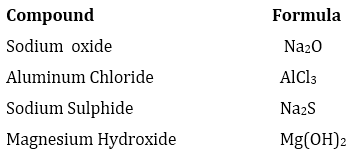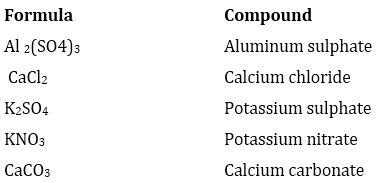### Atoms and Molecules - Solutions

CBSE class IX Science

NCERT Solutions
Chapter 3
Atoms and Molecules

(Page No. 32)

1. In a reaction, 5.3 g of sodium carbonate reacted with 6 g of ethanoic acid. The products were 2.2 g of carbon dioxide, 0.9 g water and 8.2 g of sodium ethanoate. Show that these observations are in agreement with the law of conservation of mass.

sodium carbonate + ethanoic acid → sodium ethanoate + carbon dioxide + water

Ans. According to the law of conservation of mass:

Mass of reactants = Mass of products

Let's calculate and find out both results –

Mass of reactants = Mass of sodium carbonate +Mass of ethanoic acid
= 5.3g + 6g
= 11.3g

Mass of products = Mass of sodium ethanoate + Mass of carbon dioxide + Mass of water
= 8.2g +2.2g + 0.9g = 11.3g

Hence it is proved that these observations are in agreement with the law of conservation of mass.

2.Hydrogen and oxygen combine in the ratio of 1:8 by mass to form water. What mass of oxygen gas would be required to react completely with 3 g of hydrogen gas?

Ans. As per the given 1:8 ratio mass of oxygen gas required to react completely with 1g of hydrogen gas is 8g.

Therefore, mass of oxygen gas required to react completely with 3g of hydrogen gas will be = 3 x 8 = 24g

3.Which postulate of Dalton’s atomic theory is a result of the law of conservation of mass?

Ans. The following postulate of Dalton's atomic theory is a result of the law of conservation of mass:

"Atoms are indivisible particles which cannot be created or destroyed in a chemical reaction."

4.Which postulate of Dalton’s atomic theory can explain the law of definite proportions?

Ans. The following postulate of Dalton's atomic theory can explain the law of definite proportions :

"The relative number and kinds of atoms are constant in a given compound."

(Page No. 35)

1.Define the atomic mass unit.

Ans. Atomic mass unit is defined as the mass equal to exactly one-twelfth(1/12th) of the mass of an atom of carbon-12.

2.Why is it not possible to see an atom with naked eyes?

Ans. An atom is an extremely small particle whose radius is of the order 10-10m. This size is so small that our eyes are not able to see it.

(Page No. 39)

1. Write down the formulae of

(i) sodium oxide

(ii) aluminium chloride

(iii) sodium sulphide

(iv) magnesium hydroxide

Ans.2. Write down the names of compounds represented by following formulae:

(i) Al2(SO4)3

(ii) CaCl2

(iii) K2SO4

(iv) KNO3

(v) CaCO3

Ans.3. What is meant by the term chemical formula?

Ans. A chemical formula is the representation of elements present in a compound with the help of symbols and also the number of atoms of each element with those numbers only. for eg: A molecule of water (compound) contains 2 atoms of hydrogen and one atom of oxygen hence its chemical formula is H2O.

4. How many atoms are present in a

(i) H2S molecule and

(ii) PO43- ion?

Ans. (i) 2 atoms of hydrogen + 1 atom of sulphur = 3 atoms

(ii)1 atom of phosphorus + 4 atoms of oxygen = 5 atoms

(Page No. 40)

1. Calculate the molecular masses of H2,O2,Cl2,CO2,CH4,C2H6,C2H4,NH3,CH3OH

Ans. Molecular mass of H2= atomic mass of H x 2 = 1 x 2 = 2u

Molecular mass of  O2= atomic mass of O x 2 = 16 x 2 = 32u

Molecular mass of Cl2= atomic mass of Cl x 2 = 35.5 x 2 = 71u

Molecular mass of CO2= atomic mass of C + (atomic mass of O x 2)
= 12 + (16 x 2)
= (12 + 32)
= 44 u

Molecular mass of  CH4= 12 + atomic mass of hydrogen x 4
= 12 (1 x 4)
= 12 + 4
= 16 u

Molecular mass of C2H6= 12 x 2 + 6 x 1= 24+6 = 30 u
Molecular mass of C2H4= 12 x 2 + 4 x 1=24 + 4 = 28 u
Molecular mass of NH3= 14 + 3= 17 u
Molecular mass of CH3OH= 12+1x3+16+1=12+3+16+1=32 u

2. Calculate the formula unit masses of ZnO, Na2O, K2CO3, given atomic masses of Zn = 65 u, Na = 23 u, K = 39 u, C = 12 u, and O = 16 u.

Ans. Formula unit mass of:

i) ZnO = Atomic mass of Zn + atomic mass of O = (65 + 16) u = 81 u

ii) Na2O= Atomic mass of Na + atomic mass of  O= 23 x 2 + 16= 46 + 16 = 62 u

iii) K2CO3= Atomic mass of K + atomic mass of C + atomic mass of O= 39 x 2 + 12 + 3 x 16= 78 + 12 + 48 = 138 u

(Page No. 42)

1. If one mole of carbon atoms weighs 12 grams, what is the mass (in grams) of 1 atom of carbon?

Ans. Weight of one mole of carbon = atomic mass of carbon (1 atom of carbon) = 12 u

Therefore, one mole of carbon contains = 12 g = 6.022 x 1023atoms (Avogadro number)

So, 1 atom of Carbon = $\frac{12}{6.022×{10}^{23}}$g
= 1.993 x 10-23 g

2.Which has more number of atoms, 100 grams of sodium or 100 grams of iron (given ,atomic mass of Na = 23 u, Fe = 56 u)?

Ans. We can find out the element with more number of atoms by calculating number of moles of each of them:

Number of moles of sodium in 100 g = 100/23 = 4.34
Number of moles of iron in 100g= 100/56 = 1.78
Therefore, the number of atoms is more for sodium as compared to iron.